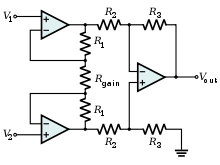# Common-mode rejection ratio

Common-mode rejection ratio

The common-mode rejection ratio (CMRR) of a differential amplifier (or other device) is the tendency of the devices to reject the input signals common to both input leads. A high CMRR is important in applications where the signal of interest is represented by a small voltage fluctuation superimposed on a (possibly large) voltage offset, or when relevant information is contained in the voltage difference between two signals. (An example is audio transmission over balanced lines.)

Ideally, a differential amplifier takes the voltages V + and V on its two inputs and produces an output voltage Vo = Ad(V +V ), where Ad is the differential gain. However, the output of a real differential amplifier is better described as$V_{\mathrm{o}} = A_\mathrm{d} (V_+ - V_-) + \tfrac{1}{2} A_\mathrm{cm} (V_+ + V_-),$

where Acm is the common-mode gain, which is typically much smaller than the differential gain.

The CMRR is defined as the ratio of the powers of the differential gain over the common-mode gain, measured in positive decibels (thus using the 20 log rule):$\mathrm{CMRR} = 10\log_{10} \left (\frac{A_\mathrm{d}}{A_\mathrm{cm}} \right)^2 = 20\log_{10} \left (\frac{A_\mathrm{d}}{|A_\mathrm{cm}|} \right)$

As differential gain should exceed common-mode gain, this will be a positive number, and the higher the better.

The CMRR is a very important specification, as it indicates how much of the common-mode signal will appear in your measurement. The value of the CMRR often depends on signal frequency as well, and must be specified as a function thereof.

It is often important in reducing noise on transmission lines. For example, when measuring the resistance of a thermocouple in a noisy environment, the noise from the environment appears as an offset on both input leads, making it a common-mode voltage signal. The CMRR of the measurement instrument determines the attenuation applied to the offset or noise.

## Example: operational amplifiersTypical instrumentation amplifier implementation, designed to have a high CMRR.

An operational amplifier (op-amp) has two inputs, V+ and V-, and an open-loop gain G. In the ideal case, the output of an ideal op-amp behaves according to the equation$V_\mathrm{out} = (V_+ - V_-) \cdot G_\mathrm{openloop}$

This equation represents an infinite CMRR: if both inputs fluctuate by the same amount (while maintaining a constant difference V+ - V-), this change will have no bearing on the output. In real applications, this is not always the case: the lower the CMRR, the larger the effect on the output signal, following the general equation$V_\mathrm{out} = (V_+ - V_-) \cdot G_\mathrm{openloop} \pm \frac {V_\mathrm{cm}}{ 10 ^ {\frac {CMRR}{20}}}$

Where VCM represents the common-mode voltage at the inputs, or (V+ + V-)/2.

The 741, a common op-amp chip, has a CMRR of 90 dB, which is reasonable in most cases. A value of 70 dB may be adequate for applications which are insensitive to the effects on amplifier output;some high-end devices may use op-amps with a CMRR of 120 dB or more.

So for example, an op-amp with 90dB CMRR operating with 10V of common-mode will have an output error of ±316uV.

Wikimedia Foundation. 2010.

Нужна курсовая?

### Look at other dictionaries:

• Common-Mode Rejection Ratio — Die Gleichtaktunterdrückung (engl. Common Mode Rejection Ratio, kurz CMRR) gibt in der Elektronik an, wie wenig sich die Ausgangsspannungs ändert, wenn sich die beiden Eingangsspannungen eines elektrischen Differenzverstärkers um den gleichen… …   Deutsch Wikipedia

• common-mode rejection ratio — sinfazinio signalo malšinimo faktorius statusas T sritis radioelektronika atitikmenys: angl. common mode rejection ratio vok. Gleichtaktunterdrückungsfaktor, m rus. коэффициент ослабления синфазного сигнала, m pranc. facteur de réjection de mode… …   Radioelektronikos terminų žodynas

• common mode rejection ratio — sinfazinės įtampos malšinimo santykis statusas T sritis Standartizacija ir metrologija apibrėžtis Įtampos, veikiančios tarp apibrėžto atskaitos taško ir dviejų vienas su kitu tam tikru būdu sujungtų įėjimo gnybtų, ir tarp įėjimo gnybtų… …   Penkiakalbis aiškinamasis metrologijos terminų žodynas

• Common mode rejection ratio — A&V • For a differential amplifier, the ratio of differential gain to common mode gain. • Expressed in dB, the ratio of common mode input voltage to output voltage. • For an operational amplifier, the ratio of the change in input/offset voltage… …   Audio and video glossary

• common-mode rejection ratio — F/A/V The ratio of the common mode interference voltage at the input of a circuit, to the corresponding interference voltage at the output …   Audio and video glossary

• Common-Mode Rejection — Die Gleichtaktunterdrückung (engl. Common Mode Rejection Ratio, kurz CMRR) gibt in der Elektronik an, wie wenig sich die Ausgangsspannungs ändert, wenn sich die beiden Eingangsspannungen eines elektrischen Differenzverstärkers um den gleichen… …   Deutsch Wikipedia

• Common mode rejection (CMR) — A&V A measure of how well a differential amplifier rejects a signal which appears simultaneously and in phase at both input terminals. As a specification, CMR is usually stated as a dB ratio at a given frequency …   Audio and video glossary

• Common mode — is a term in engineering with at least two independent meanings. Of electrical signals, Common mode rejection ratio, the ratio of rejection of common mode signals to differential signals Common mode interference, interference that appears on both …   Wikipedia

• Common-mode signal — is the component of an analog signal which is present with one sign on all considered conclusions. In electronics where the signal is transferred with differential voltage use, the common mode signal is called a half sum of voltages: When… …   Wikipedia

• Common-mode interference — In telecommunication, the term common mode interference has the following meanings: Interference that appears on both signal leads (signal and circuit return), or the terminals of a measuring circuit, and ground. A form of coherent interference… …   Wikipedia